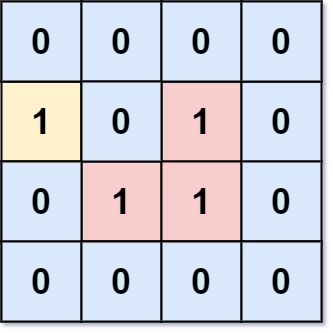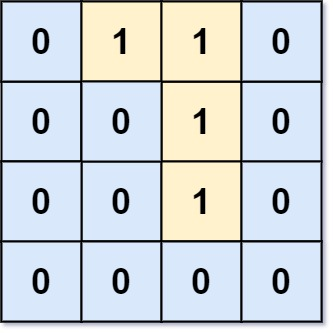## Algorithm

Problem Name: 1020. Number of Enclaves

You are given an `m x n` binary matrix `grid`, where `0` represents a sea cell and `1` represents a land cell.

A move consists of walking from one land cell to another adjacent (4-directionally) land cell or walking off the boundary of the `grid`.

Return the number of land cells in `grid` for which we cannot walk off the boundary of the grid in any number of moves.

Example 1:```Input: grid = [[0,0,0,0],[1,0,1,0],[0,1,1,0],[0,0,0,0]]
Output: 3
Explanation: There are three 1s that are enclosed by 0s, and one 1 that is not enclosed because its on the boundary.
```

Example 2:```Input: grid = [[0,1,1,0],[0,0,1,0],[0,0,1,0],[0,0,0,0]]
Output: 0
Explanation: All 1s are either on the boundary or can reach the boundary.
```

Constraints:

• `m == grid.length`
• `n == grid[i].length`
• `1 <= m, n <= 500`
• `grid[i][j]` is either `0` or `1`.

## Code Examples

### #1 Code Example with Java Programming

```Code - Java Programming```

``````
class Solution {
private static final int[][] DIRS = {{1, 0}, {0, 1}, {-1, 0}, {0, -1}};

public int numEnclaves(int[][] grid) {
int result = 0;
for (int i = 0; i < grid.length; i++) {
for (int j = 0; j < grid.length; j++) {
result += grid[i][j];
if (i * j == 0 || i == grid.length - 1 || j == grid[i].length - 1) {
}
}
}
while (!queue.isEmpty()) {
int[] removed = queue.remove();
int x = removed;
int y = removed;
if (x < 0 || y < 0 || x == grid.length || y == grid.length || grid[x][y] != 1) {
continue;
}
grid[x][y] = 0;
result--;
for (int[] dir : DIRS) {
queue.add(new int[]{x + dir, y + dir});
}
}
return result;
}
}
``````
Copy The Code &

Input

cmd
grid = [[0,0,0,0],[1,0,1,0],[0,1,1,0],[0,0,0,0]]

Output

cmd
3

### #2 Code Example with Javascript Programming

```Code - Javascript Programming```

``````
const numEnclaves = function(A) {
let res = 0
const dirs = [[-1, 0], [1, 0], [0, 1], [0, -1]]
const visited = Array.from({ length: A.length }, () =>
new Array(A.length).fill(false)
)
for (let row = 0; row < A.length; row++) {
for (let col = 0; A && col < A.length; col++) {
if (
(row === 0 ||
col === 0 ||
row === A.length - 1 ||
col === A.length - 1) &&
A[row][col] === 1
) {
dfs(A, row, col, visited, dirs)
}
}
}
for (let row = 0; row < A.length; row++) {
for (let col = 0; A && col < A.length; col++) {
if (A[row][col] === 1) {
res += 1
}
}
}
return res
}

function dfs(A, row, col, v, dirs) {
if (
row < 0 ||
row >= A.length ||
col < 0 ||
col >= A.length ||
v[row][col] ||
A[row][col] === 0
)
return

v[row][col] = true
A[row][col] = 0

for (let dir of dirs) {
dfs(A, row + dir, col + dir, v, dirs)
}
}
``````
Copy The Code &

Input

cmd
grid = [[0,0,0,0],[1,0,1,0],[0,1,1,0],[0,0,0,0]]

Output

cmd
3

### #3 Code Example with Python Programming

```Code - Python Programming```

``````
class Solution:
def numEnclaves(self, A: List[List[int]]) -> int:
def dfs(i, j):
A[i][j] = 0
for x, y in (i - 1, j), (i + 1, j), (i, j - 1), (i, j + 1):
if 0 <= x < m and 0 <= y < n and A[x][y]:
dfs(x, y)
m, n = len(A), len(A)
for i in range(m):
for j in range(n):
if A[i][j] == 1 and (i == 0 or j == 0 or i == m - 1 or j == n - 1):
dfs(i, j)
return sum(sum(row) for row in A)
``````
Copy The Code &

Input

cmd
grid = [[0,1,1,0],[0,0,1,0],[0,0,1,0],[0,0,0,0]]